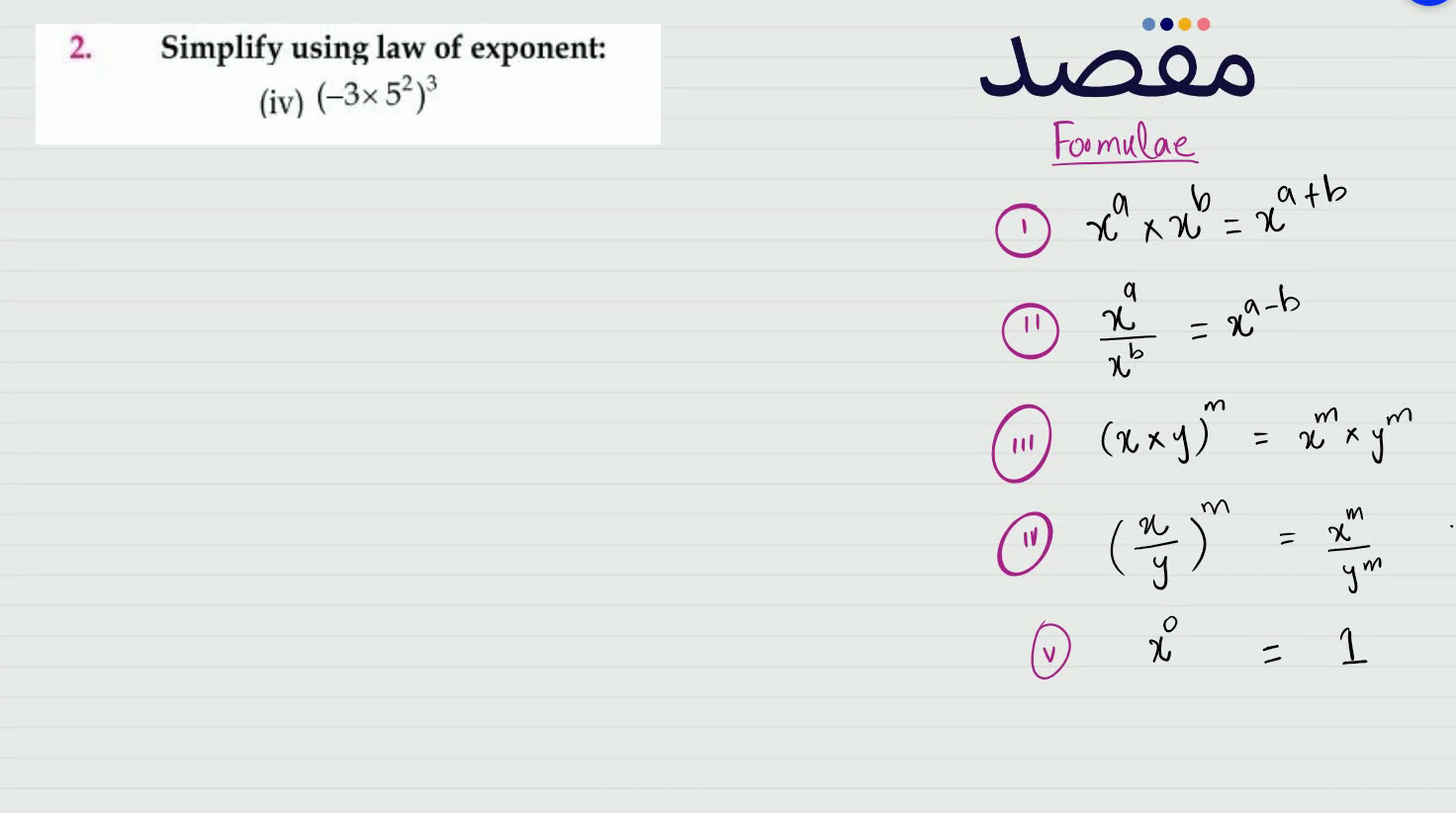# Classes

Change the way you learn with Maqsad's classes. Local examples, engaging animations, and instant video solutions keep you on your toes and make learning fun like never before!

Class 9Class 10First YearSecond Year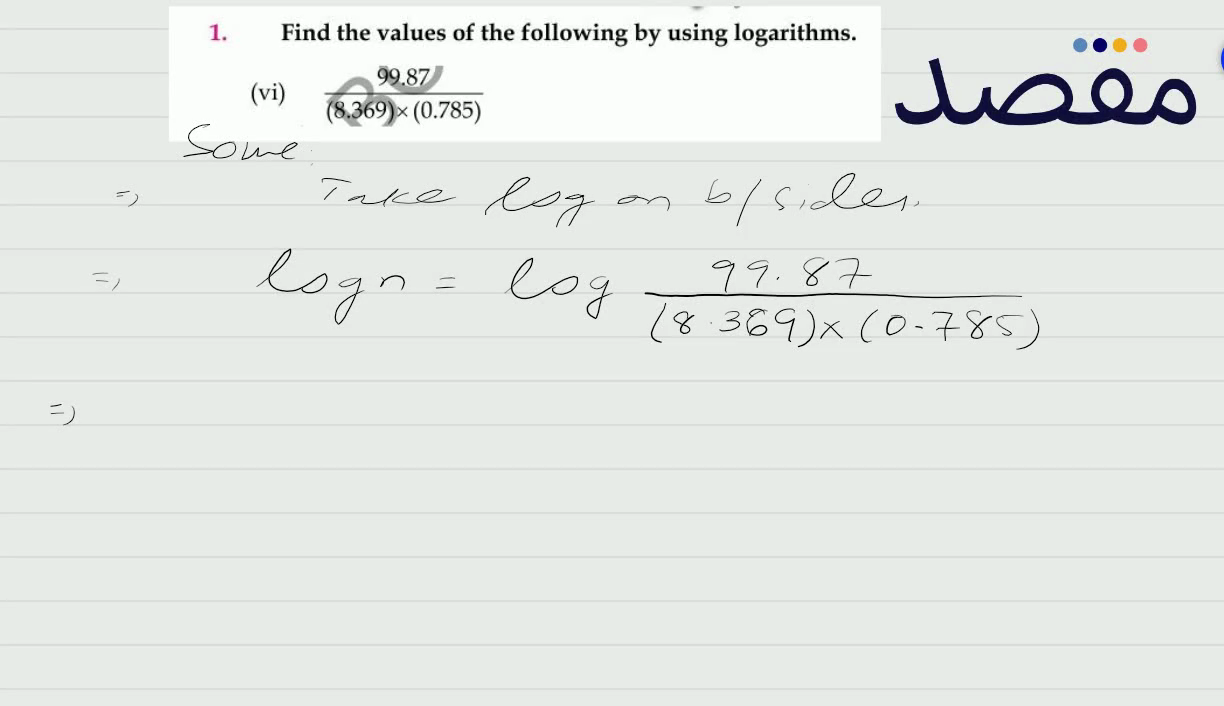### 1. Identify the following statements as true or false.(vi) If (a-1)-(b+3) i=5+8 i then a=6 and b=-11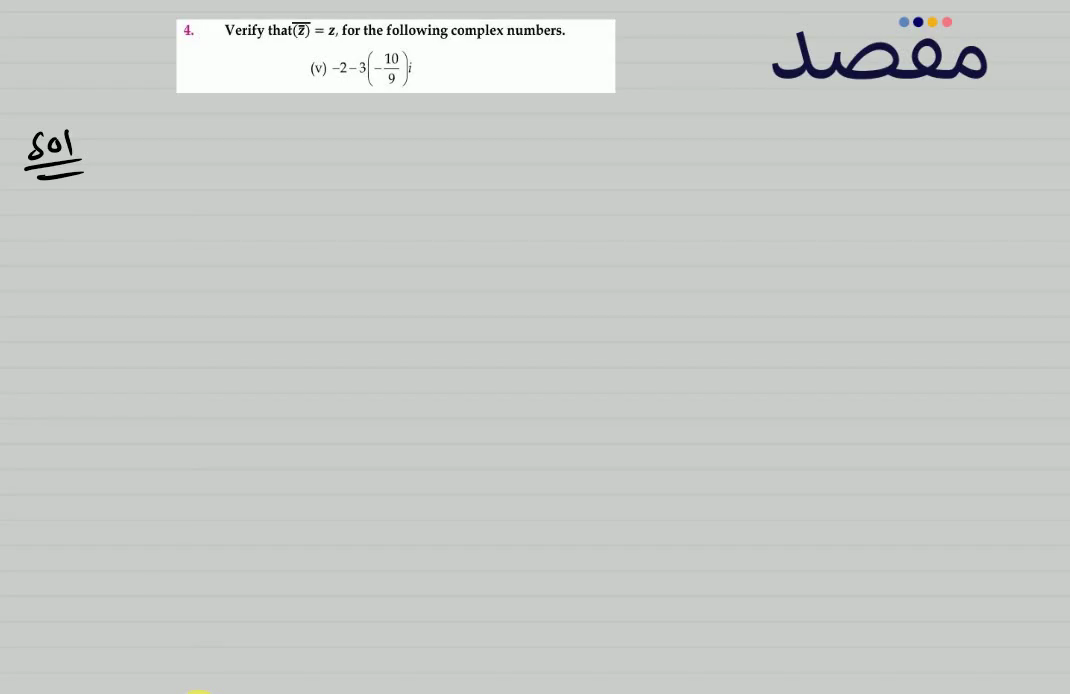### 4. Verify that (\overline{\bar{z}})=z for the following complex numbers.(v) -2-3\left(-\frac{10}{9}\right) i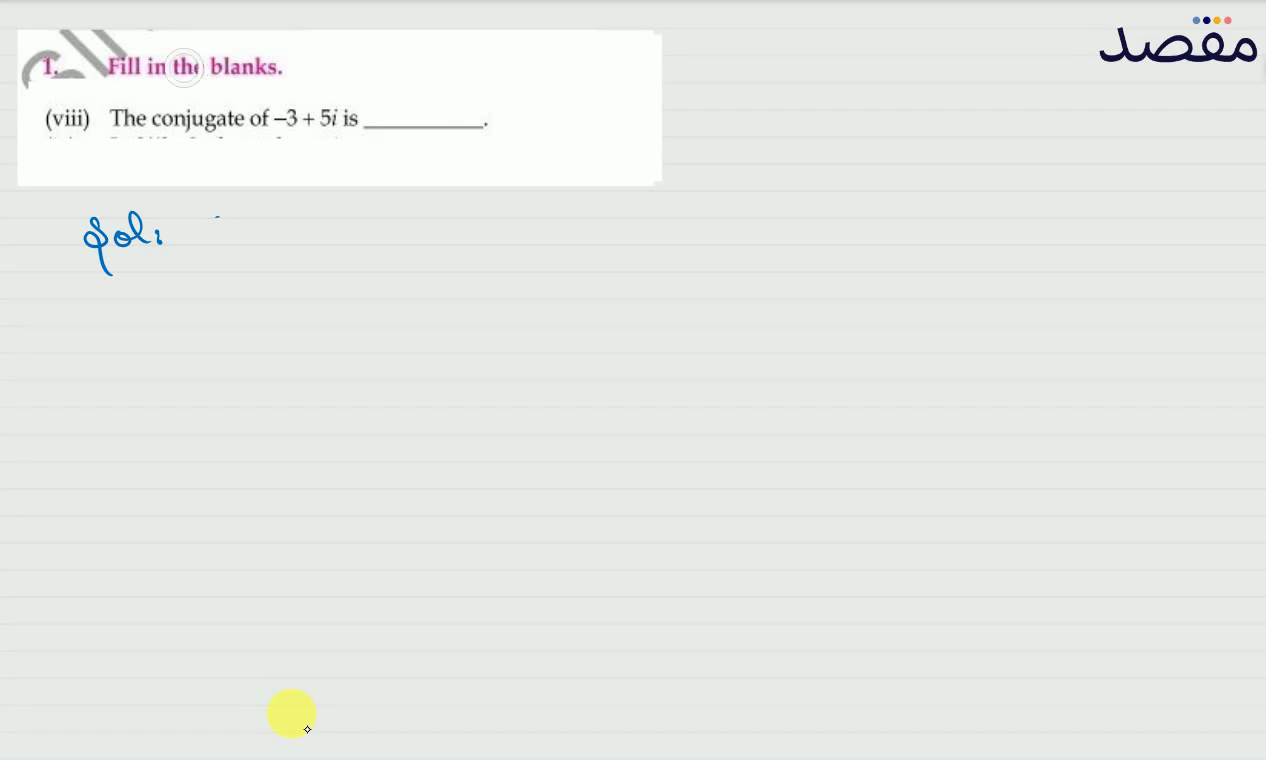### 1. Fill in the blanks.(viii) The conjugate of -3+5 i is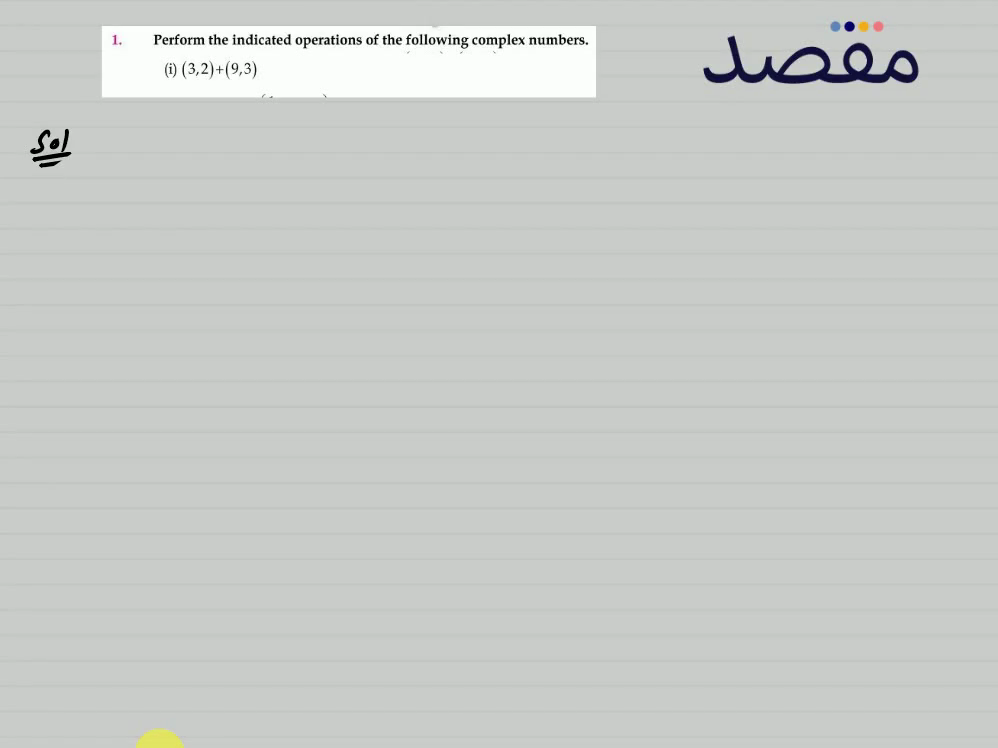### 1. Perform the indicated operations of the following complex numbers.(i) (32)+(93)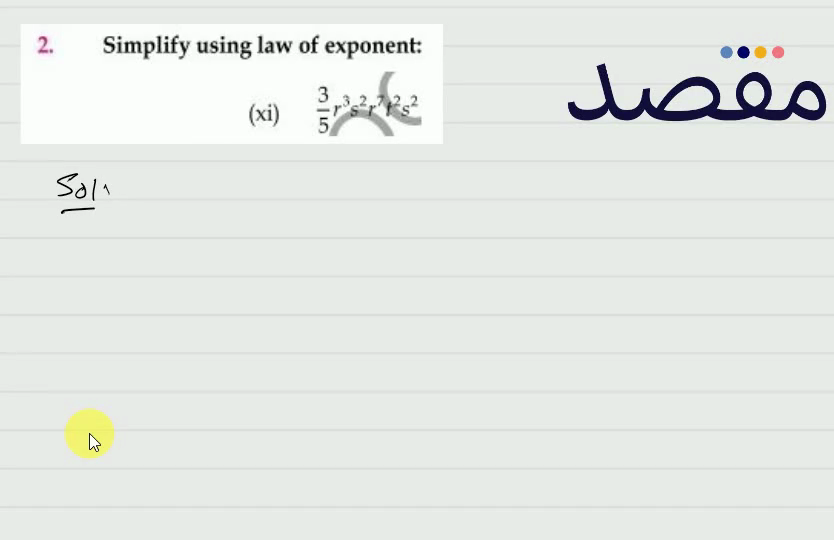### 2. Simplify using law of exponent:(xi) \frac{3}{5} r^{3} s^{2} r^{7} r^{2} s^{2}